Test: IIR Filter Design

# Test: IIR Filter Design

Test Description

## 10 Questions MCQ Test Signals and Systems | Test: IIR Filter Design

Test: IIR Filter Design for Electrical Engineering (EE) 2023 is part of Signals and Systems preparation. The Test: IIR Filter Design questions and answers have been prepared according to the Electrical Engineering (EE) exam syllabus.The Test: IIR Filter Design MCQs are made for Electrical Engineering (EE) 2023 Exam. Find important definitions, questions, notes, meanings, examples, exercises, MCQs and online tests for Test: IIR Filter Design below.
Solutions of Test: IIR Filter Design questions in English are available as part of our Signals and Systems for Electrical Engineering (EE) & Test: IIR Filter Design solutions in Hindi for Signals and Systems course. Download more important topics, notes, lectures and mock test series for Electrical Engineering (EE) Exam by signing up for free. Attempt Test: IIR Filter Design | 10 questions in 10 minutes | Mock test for Electrical Engineering (EE) preparation | Free important questions MCQ to study Signals and Systems for Electrical Engineering (EE) Exam | Download free PDF with solutions
 1 Crore+ students have signed up on EduRev. Have you?
Test: IIR Filter Design - Question 1

### In IIR Filter design by the Bilinear Transformation, the Bilinear Transformation is a mapping from

Detailed Solution for Test: IIR Filter Design - Question 1

Explanation: From the equation,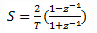it is clear that transformation occurs from s-plane to z-plane

Test: IIR Filter Design - Question 2

### In Bilinear Transformation, aliasing of frequency components is been avoided.

Detailed Solution for Test: IIR Filter Design - Question 2

Explanation: The bilinear transformation is a conformal mapping that transforms the jΩ-axis into the unit circle in the z-plane only once, thus avoiding the aliasing.

Test: IIR Filter Design - Question 3

### Is IIR Filter design by Bilinear Transformation is the advanced technique when compared to other design techniques?

Detailed Solution for Test: IIR Filter Design - Question 3

Explanation: Because in other techniques, only lowpass filters and limited class of bandpass filters are been supported. But this technique overcomes the limitations of other techniques and supports more.

Test: IIR Filter Design - Question 4

The approximation of the integral in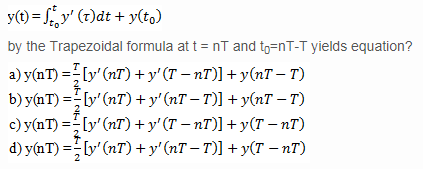Detailed Solution for Test: IIR Filter Design - Question 4

Explanation: By integrating the equation,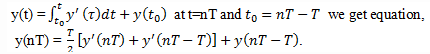Test: IIR Filter Design - Question 5

We use y'(nT)=-ay(nT)+bx(nT) to substitute for the derivative in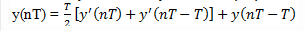and thus obtain a difference equation for the equivalent discrete-time system. With y(n) = y(nT ) and x(n) = x( nT), we obtain the result as _ of the following?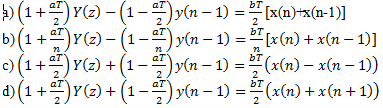Detailed Solution for Test: IIR Filter Design - Question 5

Explanation: When we substitute the given equation in the derivative of other we get the resultant required equation.

Test: IIR Filter Design - Question 6

The z-transform of below difference equation is?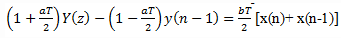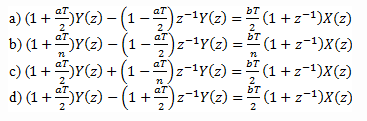Detailed Solution for Test: IIR Filter Design - Question 6

Explanation: By performing the z-transform of the given equation, we get the required output/equation.

Test: IIR Filter Design - Question 7

What is the system function of the equivalent digital filter? H(z) = Y(z)/X(z ) = ?

Detailed Solution for Test: IIR Filter Design - Question 7

Explanation: As we considered analog linear filter with system function H(s) = b/s+a
Hence, we got an equivalent system function
where,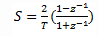Test: IIR Filter Design - Question 8

In the Bilinear Transformation mapping, which of the following are correct?

Detailed Solution for Test: IIR Filter Design - Question 8

Explanation: The bilinear transformation is a conformal mapping that transforms the jΩ-axis into the unit circle in the z-plane and all the points are linked as mentioned above.

Test: IIR Filter Design - Question 9

In Nth order differential equation, the characteristics of bilinear transformation, let z= rejw,s=o+jΩ Then for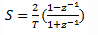the values of Ω, ℴ are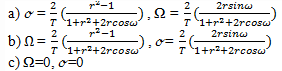d) None

Detailed Solution for Test: IIR Filter Design - Question 9

Explanation: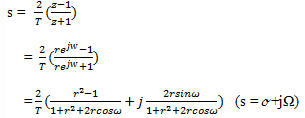Test: IIR Filter Design - Question 10

In equation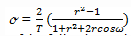if r < 1 then ℴ < 0 and then mapping from s-plane to z-plane occurs in which of the following order?

Detailed Solution for Test: IIR Filter Design - Question 10

Explanation: In the above equation, if we substitute the values of r, ℴ then we get mapping in the required way

## Signals and Systems

32 videos|76 docs|63 tests
 Use Code STAYHOME200 and get INR 200 additional OFF Use Coupon Code
Information about Test: IIR Filter Design Page
In this test you can find the Exam questions for Test: IIR Filter Design solved & explained in the simplest way possible. Besides giving Questions and answers for Test: IIR Filter Design, EduRev gives you an ample number of Online tests for practice

## Signals and Systems

32 videos|76 docs|63 tests